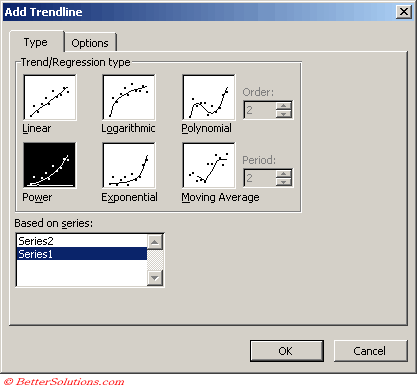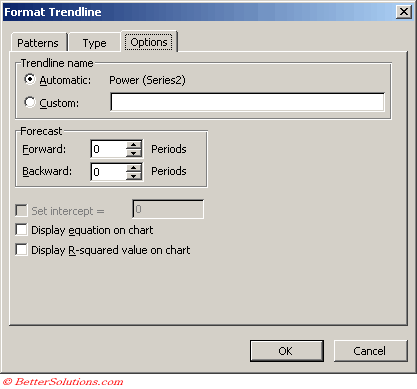# Power

Creates a curved line to compare measurements that increase at a specific rate.
This trendline is not available if the data series contains any zero or negative numbers
This can only be used with positive values.
A power trendline is a curved line that is used with data sets that compare measurements that increase at a specific rate for example, the acceleration of a race car at 1-second intervals.
You cannot create a power trendline if your data contains zero or negative values.

A power trendline by using the following equation to calculate the least squares fit through points
y=cxb
where c and b are constants
Note: The option is not available when your data includes negative or zero values

Equation: y=c*x^b
c: =EXP(INDEX(LINEST(LN(y),LN(x),,),1,2))
b: =INDEX(LINEST(LN(y),LN(x),,),1)